Updating search results...

# 37 Results

View
Selected filters:
• MI.Math.Content.5.NF.A.1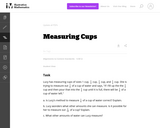Unrestricted Use
CC BY
Rating
0.0 stars

This is a task from the Illustrative Mathematics website that is one part of a complete illustration of the standard to which it is aligned. Each task has at least one solution and some commentary that addresses important asects of the task and its potential use. Here are the first few lines of the commentary for this task: Lucy has measuring cups of sizes 1 cup, $\frac{1}{2}$ cup, $\frac{1}{3}$ cup, and $\frac{1}{4}$ cup. She is trying to measure out $\frac{1}{6}$ of a cu...

Subject:
Mathematics
Material Type:
Activity/Lab
Provider:
Illustrative Mathematics
Provider Set:
Illustrative Mathematics
Author:
Illustrative Mathematics
08/07/2013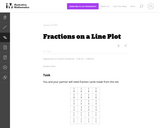Unrestricted Use
CC BY
Rating
0.0 stars

This is a task from the Illustrative Mathematics website that is one part of a complete illustration of the standard to which it is aligned. Each task has at least one solution and some commentary that addresses important asects of the task and its potential use. Here are the first few lines of the commentary for this task: You and your partner will need fraction cards made from this set: Label the line-plot below with $\frac18$'s. Cut out and divide the cards evenly betwe...

Subject:
Education
Mathematics
Material Type:
Activity/Lab
Provider:
Illustrative Mathematics
Provider Set:
Illustrative Mathematics
Author:
Illustrative Mathematics
11/05/2013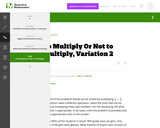Unrestricted Use
CC BY
Rating
0.0 stars

This is a task from the Illustrative Mathematics website that is one part of a complete illustration of the standard to which it is aligned. Each task has at least one solution and some commentary that addresses important aspects of the task and its potential use.

Subject:
Mathematics
Ratios and Proportions
Material Type:
Activity/Lab
Provider:
Illustrative Mathematics
Provider Set:
Illustrative Mathematics
Author:
Illustrative Mathematics
02/16/2018Conditional Remix & Share Permitted
CC BY-NC-SA
Rating
0.0 stars

This short video and interactive assessment activity is designed to teach fifth graders about adding and subtracting fractions with different denominators.

Subject:
Mathematics
Numbers and Operations
Material Type:
Assessment
Interactive
Lecture
Provider:
CK-12 Foundation
Provider Set:
CK-12 Elementary Math
04/03/2018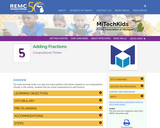Rating
0.0 stars

The math learning center is an app and online platform that allows students to use manipulatives virtually. In this activity, students will use virtual manipulatives to add fractions.

Subject:
Education
Educational Technology
Material Type:
Activity/Lab
Game
Interactive
Module
Provider:
REMC Association of Michigan
Author:
REMC Association of Michigan
03/16/2019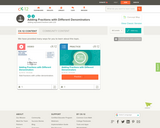Conditional Remix & Share Permitted
CC BY-NC-SA
Rating
0.0 stars

This short video and interactive assessment activity is designed to teach fifth graders about adding fractions with different denominators.

Subject:
Mathematics
Numbers and Operations
Material Type:
Assessment
Interactive
Lecture
Provider:
CK-12 Foundation
Provider Set:
CK-12 Elementary Math
04/03/2018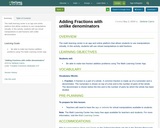Unrestricted Use
CC BY
Rating
0.0 stars

The math learning center is an app and online platform that allows students to use manipulatives virtually. In this activity, students will use virtual manipulatives to add fractions with unlike denominators

Subject:
Mathematics
Material Type:
Activity/Lab
08/28/2020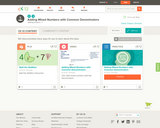Conditional Remix & Share Permitted
CC BY-NC-SA
Rating
0.0 stars

This short video and interactive assessment activity is designed to teach fifth graders about adding mixed numbers with common denominators.

Subject:
Mathematics
Numbers and Operations
Material Type:
Assessment
Interactive
Lecture
Provider:
CK-12 Foundation
Provider Set:
CK-12 Elementary Math
04/03/2018Unrestricted Use
CC BY
Rating
0.0 stars

This Nrich problem embeds adding and subtracting fractions into a bigger problem to increase interest.

Subject:
Mathematics
Material Type:
Activity/Lab
06/17/2021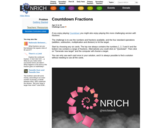Unrestricted Use
CC BY
Rating
0.0 stars

Countdown Fractions in this Nrich game offers a motivating context in which to practice calculating with fractions. There is usually more than one way of hitting the target, which offers an opportunity for rich discussion on the merits of alternative methods.

Subject:
Mathematics
Material Type:
Activity/Lab
Game
Interactive
06/17/2021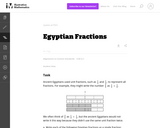Unrestricted Use
CC BY
Rating
0.0 stars

One goal of this task is to help students develop comfort and ease with adding fractions with unlike denominators. Another goal is to help them develop fraction number sense by having students decompose fractions. Because the Egyptians represented fractions differently than we do, it can also help students understand that there can be many ways of representing the same number.

Subject:
Mathematics
Numbers and Operations
Material Type:
Activity/Lab
Provider:
Illustrative Mathematics
Provider Set:
Illustrative Mathematics
Author:
Illustrative Mathematics
08/09/2012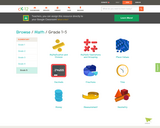Conditional Remix & Share Permitted
CC BY-NC-SA
Rating
0.0 stars

CK-12's Grade 5 Elementary Math is a series of videos and interactive videos designed to teach basic math concepts to 5th graders. Concepts covered include Multiplication and Division, Place Value, Multiple Operations and Grouping, Decimals, Time, Fractions, Money, Measurement, and Geometry.

Subject:
Mathematics
Material Type:
Activity/Lab
Interactive
Lecture
Provider:
CK-12 Foundation
Provider Set:
CK-12 Elementary Math
04/03/2018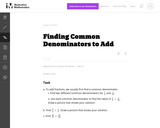Unrestricted Use
CC BY
Rating
0.0 stars

This task asks students to find and use two different common denominators to add the given fractions. The purpose of this question is to help students realize that they can use any common denominator to find a solution, not just the least common denominator.

Subject:
Mathematics
Numbers and Operations
Material Type:
Activity/Lab
Provider:
Illustrative Mathematics
Provider Set:
Illustrative Mathematics
Author:
Illustrative Mathematics
11/11/2012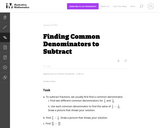Unrestricted Use
CC BY
Rating
0.0 stars

This task asks students to use two different denominators to subtract fractions. The purpose of this is to help students realize that any common denominator will work, not just the least common denominator.

Subject:
Mathematics
Numbers and Operations
Material Type:
Activity/Lab
Provider:
Illustrative Mathematics
Provider Set:
Illustrative Mathematics
Author:
Illustrative Mathematics
08/01/2012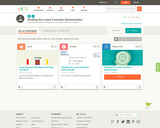Conditional Remix & Share Permitted
CC BY-NC-SA
Rating
0.0 stars

This short video and interactive assessment activity is designed to teach fifth graders about finding the least common denominator.

Subject:
Mathematics
Numbers and Operations
Material Type:
Assessment
Interactive
Lecture
Provider:
CK-12 Foundation
Provider Set:
CK-12 Elementary Math
04/03/2018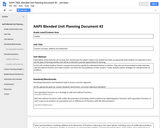Unrestricted Use
CC BY
Rating
0.0 stars

In this unit, students build on fraction concepts from previous grades to understand fractions as division. They also use visual models to make estimates, add and subtract fractions and mixed numbers, and check the reasonableness of their answers. Finally, students explore strategies for solving fraction-of problems.

Subject:
Mathematics
Material Type:
Unit of Study
Provider:
Michigan Virtual
Author:
James Keen
08/26/2016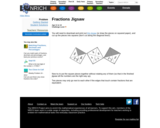Unrestricted Use
CC BY
Rating
0.0 stars

This Nrich problem provides an opportunity to find equivalent fractions and carry out some simple additions and subtractions of fractions in a context that may challenge and motivate students.

Subject:
Mathematics
Material Type:
Activity/Lab
Game
06/17/2021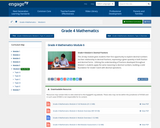Conditional Remix & Share Permitted
CC BY-NC-SA
Rating
0.0 stars

This 20-day module gives students their first opportunity to explore decimal numbers via their relationship to decimal fractions, expressing a given quantity in both fraction and decimal forms.  Utilizing the understanding of fractions developed throughout Module 5, students apply the same reasoning to decimal numbers, building a solid foundation for Grade 5 work with decimal operations.

Subject:
Mathematics
Ratios and Proportions
Material Type:
Module
Provider:
New York State Education Department
Provider Set:
EngageNY
02/01/2014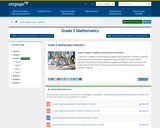Conditional Remix & Share Permitted
CC BY-NC-SA
Rating
0.0 stars

In Module 3, students' understanding of addition and subtraction of fractions extends from earlier work with fraction equivalence and decimals. This module marks a significant shift away from the elementary grades' centrality of base ten units to the study and use of the full set of fractional units from Grade 5 forward, especially as applied to algebra.

Subject:
Mathematics
Ratios and Proportions
Material Type:
Module
Provider:
New York State Education Department
Provider Set:
EngageNY
11/27/2012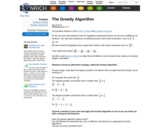Unrestricted Use
CC BY
Rating
0.0 stars

This Nrich problem follows on from Keep It Simple and Egyptian Fractions
These three problems together offer students an opportunity to engage with some mathematical ideas in depth and not just with the rather mechanical process of adding and subtracting fractions.

This problem in particular requires students to compare fractions and may deepen their understanding of their relative sizes.

Subject:
Mathematics
Material Type:
Activity/Lab